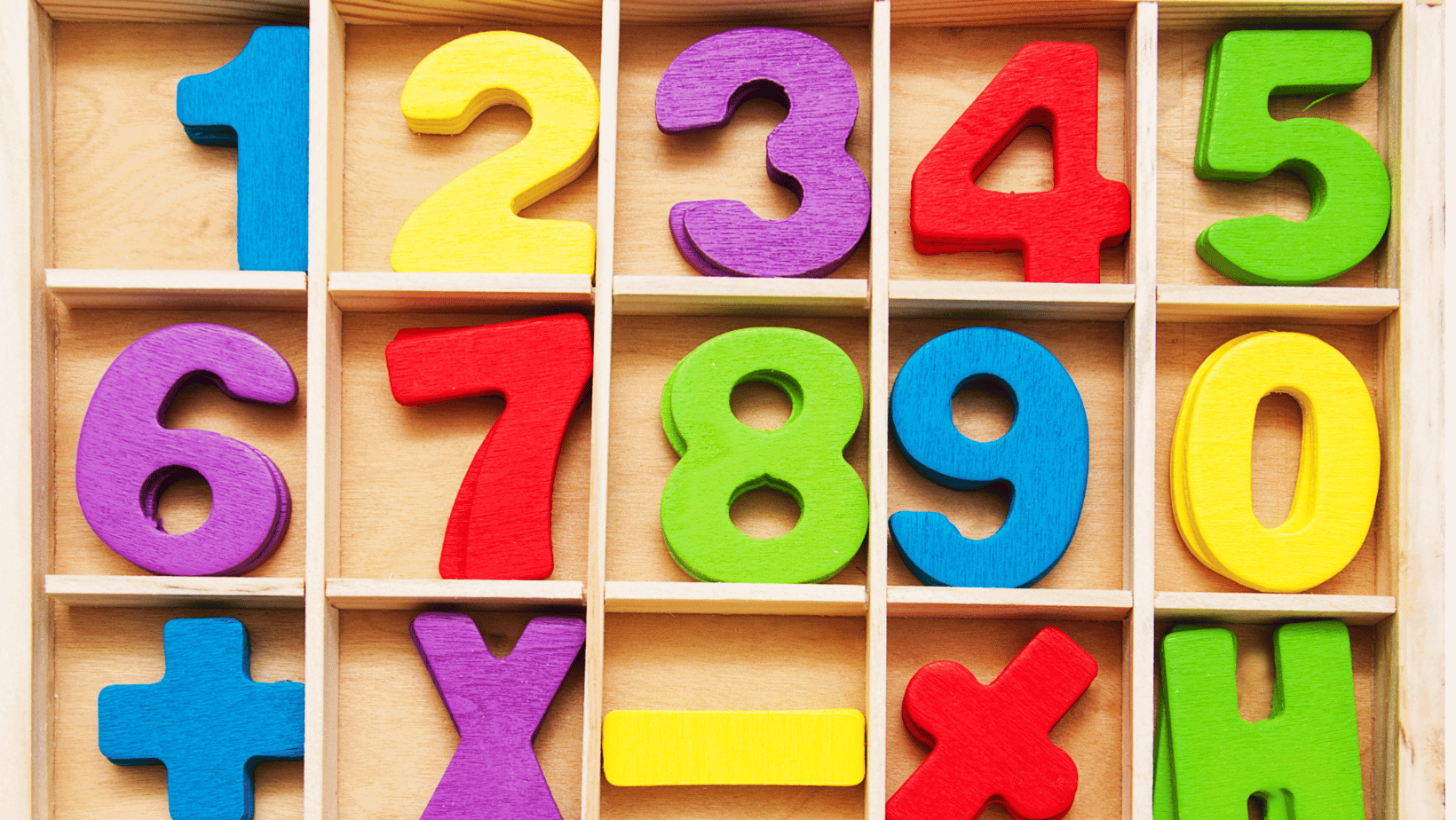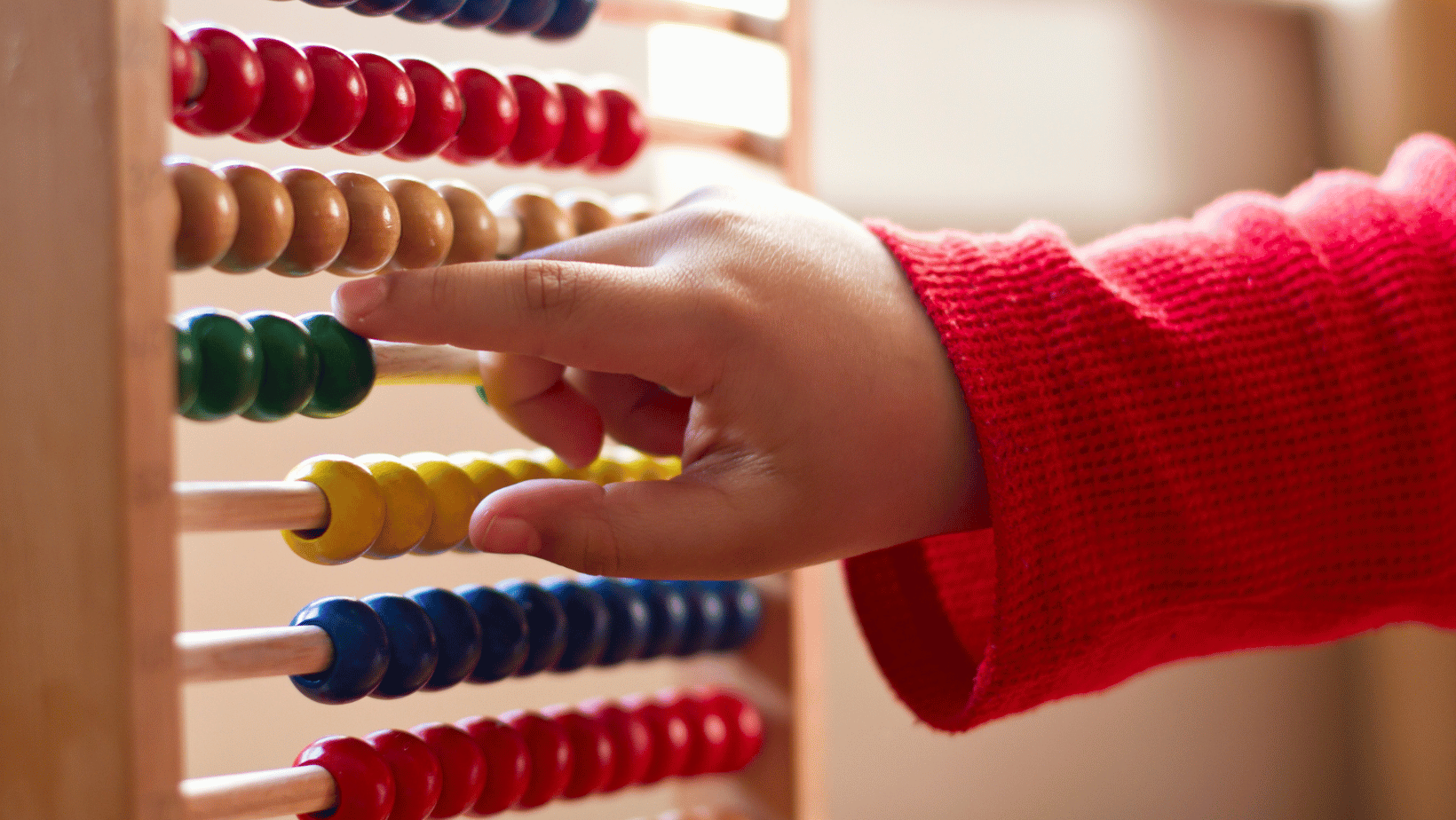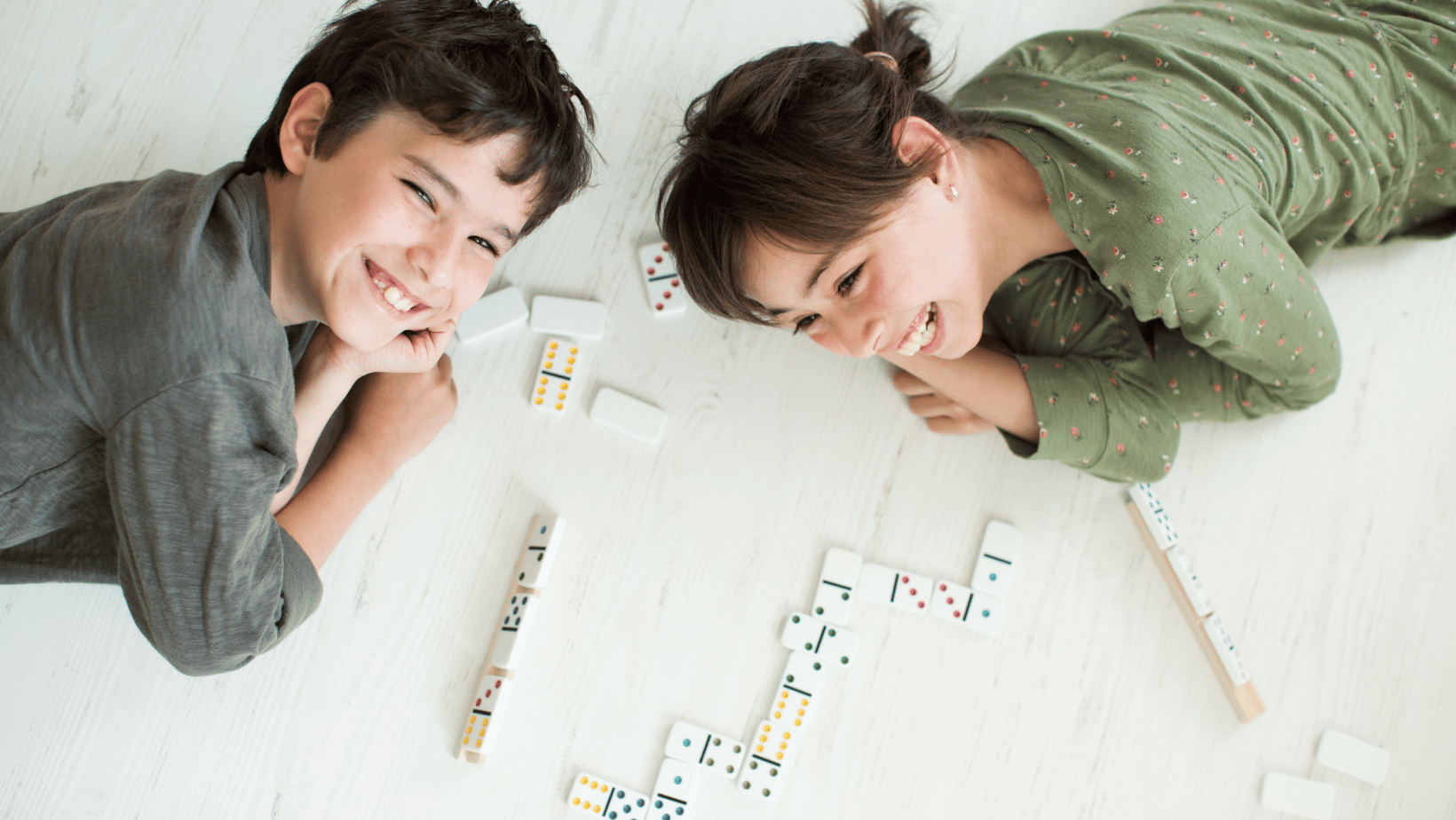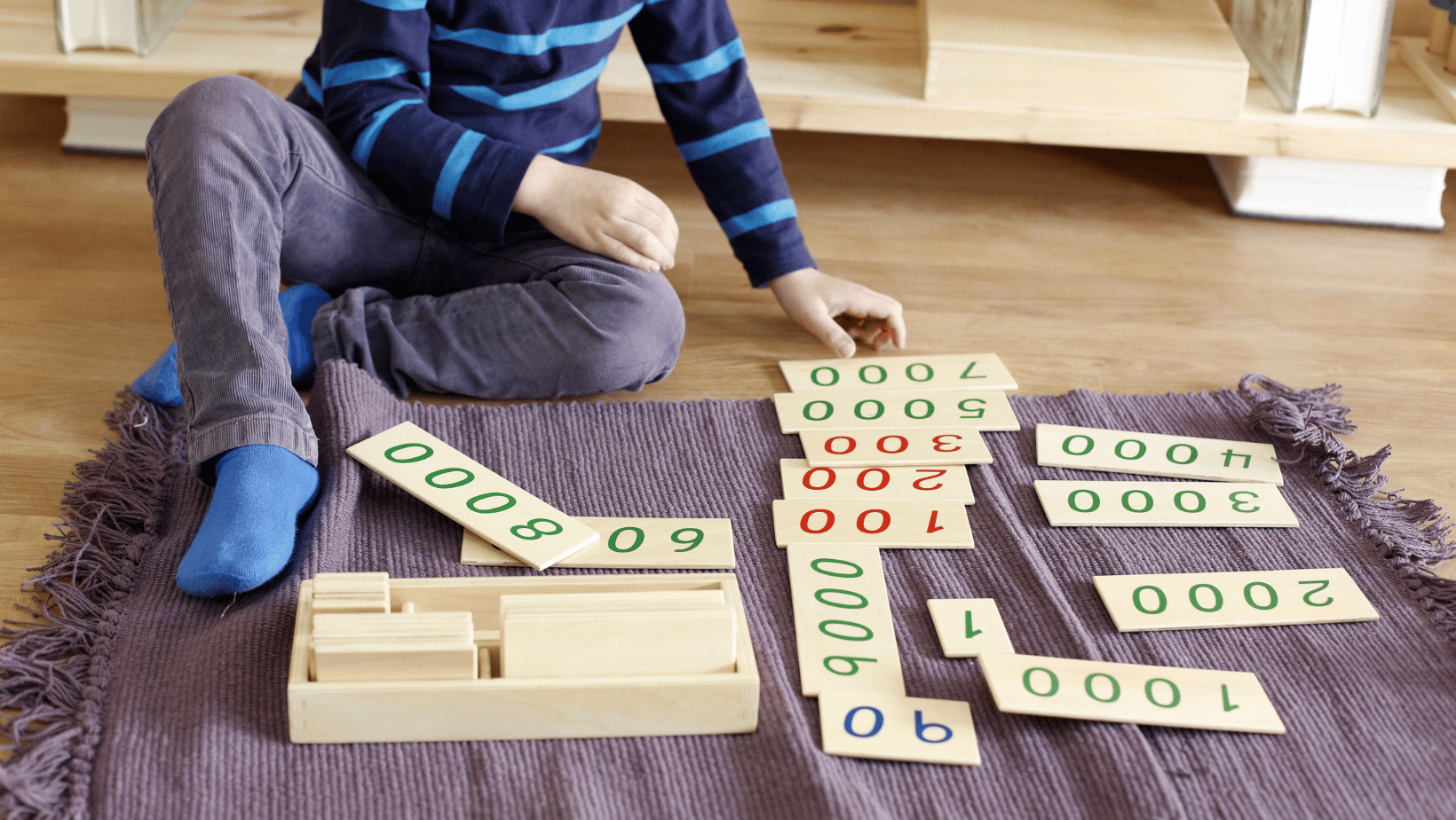# Top tips on How to Teach Geometry to Kids Easily – 2023 Guide

Do you have a child struggling to learn geometry? Don’t worry, geometry isn’t as difficult as it seems. Here are a few tips to teach your child the basics of geometry and its many shapes. You’ll be surprised at how quickly your child will pick up these new concepts which you’ll learn in this article on how to teach Geometry to kids.

## How to Teach Geometry to Kids

Geometry is an interesting and fascinating branch of mathematics that can bring great joy and fulfilment to those who learn to use it effectively. This introduction is designed to give children an opportunity to explore the basic concepts, principles, and techniques of geometry while having fun in the process.

The main idea behind the need to teach geometry to kids is to familiarize them with shapes and how they relate to each other. To do this, focus on introducing fundamental concepts such as points, lines, angles, polygons, circles, perimeters and areas. Using hands-on activities such as drawing different shapes or working with pattern blocks are visual demonstrations that help kids understand these terms in a tangible way.

To engage kids further in their geometric exploration, introduce the concept of spatial relationships by having them think about measurements. Measurement can include utilizing a ruler or protractor for calculating perimeter or area measurements or manipulating 3-dimensional objects such as paper shapes so that they fit together better from specific angles – all activities which allow children to explore geometry in an interactive way.

In addition, working with mapping activities can involve both formula calculations as well recognizing surrounding features on earth or on a map which allows for further exploration of geometric principles!

## Explaining Basic Geometric Shapes

Geometry can be one of the most challenging topics for kids to understand, but it’s also an important subject for helping children refine their analytical, spatial and problem-solving skills. Understanding basic geometric shapes is a great way to introduce your child to geometry.

Begin by providing a visual aid of each basic geometric shape: circle, triangle and square. Using objects from around the house or drawing examples on paper can help kids get comfortable with these shapes. After introducing basic geometric shapes, point out everyday objects that take on those same shapes and explain why they are shaped in such a way—a streetlight may be triangular because it provides equal lines of light in three directions or a soccer ball may be round so it rolls more easily.

Discuss how many sides and angles each basic geometric shape has; draw diagrams if needed. Explain that circle has no angles or sides, triangle has three angles and three sides while square has four angles and four sides. Be sure to review these concepts frequently until they are understood clearly by children—regular practice will reinforce their knowledge of geometry terminology and help them identify other figures with similar characteristics as they come across them in math class or real life situations.

## Exploring Angles and Lines

For students who are just beginning to explore geometry, learning about angles and lines is a great way to start. Students can explore basic concepts such as acute, right and obtuse angles by using manipulatives such as pattern blocks or angle blocks. They can also begin to learn about basic line segments, ray angles, parallel and perpendicular lines by drawing them on paper or using manipulative models.

The next step for students is to move from learning the definitions of angles and lines to understanding the relationship between them. This can be done through visualizing their properties on paper or learning the formulas that describe their properties on a graph.

Once these basics are grasped, more abstract concepts can be taught such as angle pair relationships (alternate interior angles, corresponding angles), triangle relationships (isosceles triangle properties, exterior angles of a triangle) and quadrilateral relationships (diagonals of a parallelogram bisect each other).

Students should also practice finding the measurements of unknown figure components using algebraic methods. This includes applying the Pythagorean theorem or finding missing line segments in an inscribed or circumscribed figure. By repeatedly engaging with problems like these, students will be able to better understand how line segment lengths and angle measurements are related and how they affect each other in an equation/figure.

With guidance from their teacher as well as consistent practice with developing geometric skills, students will be able to gain a comprehensive view of geometry concepts.

As you teach geometry to kids, it is important to break down concepts into manageable pieces. Introducing each individual shape and discussing its structure and properties can help children understand their mutual relationships and the larger concept of geometry overall.

Triangles are one of the most basic shapes and can be used to introduce the basics of geometry to children. They can help kids understand angled lines and measure angles, as well as recognize different types of triangles by their sides: equilateral (all three sides are equal in length), isosceles (only two sides are equal), scalene (all sides are different lengths), and right triangles (only one 90-degree angle).

These concepts can be supplemented with worksheets that allow children to name different triangles by looking at them.

Quadrilaterals are four-sided shapes which include the rectangle, square, parallelogram, trapezoid, and rhombus. These shapes can also be used to introduce math basics like recognizing types of angles (right angles, acute angles) or advanced math like finding area formulas. Worksheets that focus on identifying various figures can help children further understand these relationships.

Using visual aids such as labelled diagrams or models made from building blocks or paper cut-outs gives kids a hands-on approach for understanding geometric shapes. Practical activities such as drawing in a grid on graph paper or trying out calculations with equations helps cement the concept in their minds while making learning fun!

## Exploring Symmetry and Tessellations

Exploring symmetry and tessellations can help children understand the fundamental concepts of geometry. Through activities such as drawing patterns and shapes, children can discover the properties of different geometric figures.

• Exploring Symmetry: By examining pictures and drawing symmetrical figures, kids will begin to grasp the basic concept of symmetry: that something can be “mirror” reflected across an axis. Kids will learn how to draw lines of symmetry and identify mirrored objects.
• Tessellations: Tessellations are tiled patterns in which each shape fits perfectly together without gaps or overlaps. Kids can explore these shapes by connecting paper pieces and creating their own designs with markers or crayons. This helps them learn important concepts such as angles, coordinates, rotations, dilations, and other trigonometric expressions.

These activities build an understanding and appreciation for the beautiful order of geometry through creative play – something that will stay with children for many years to come!

## Working with 3D Shapes

Three-dimensional shapes are important for children to learn as they begin to understand the world around them. As teachers, it is our job to explain the properties of 3D shapes, such as triangular prisms, and cones. Here are a few tips for working with 3D shapes:

1. Start with definitions: Explain what a 3-dimensional shape is, and then define all basic vocabulary such as plane, vertices, faces and edges.
2. Pick objects to help children visualize 3D shapes: Use real objects such as cardboard boxes that can be taken apart or different types of balls or cubes built out of legos so they can see how they differ from one another.
3. Show how 2D shapes create 3D forms: For example, a cube is made up of six squares – this will help kids understand that these forms have flat sides which creates an enclosed volume when fitted together in the right way. Additionally, show them pieces cut out from larger shapes like rectangles and squares in order to form other 3 dimensional figures like a cube or a pyramid.
4. Have children construct their own geometrical patterns and predictions: This can include building with clay or drawing diagrams on paper –then get them to discuss their results and interpret why certain objects look like certain 3d figures based on the definition given in step 1.
5. Introduce activities for more practice: Provide activities such as completing matching puzzles or solving mazes which require kids to distinguish between different types of geometric figures in order to complete their tasks – this will help reinforce your students’ understanding of the material taught earlier in class!

## Introducing Measurement and Area

As you teach geometry to kids, introducing measurement and area is a necessary foundational skill. Start by helping them to understand how to measure lengths and distances accurately with rulers, then move onto more complex two-dimension shapes such as triangles, four sided shapes (including squares, rectangles and parallelograms), circles, donuts (or annulus) and stars. Provide visual illustrations so that they can begin to understand the relationships between different types of shapes.

Move onto area calculations by providing concrete examples such as walls, carpets or gardens that cover specific areas. Talk about the difference between perimeter measurements – lengths around an object – and area measurements which are a flat measurement across the surface of an object. Show examples of applications in science, engineering or sport that involve area calculation – this will help children make meaningful connections as it becomes relevant in their everyday lives.

## Conclusion

To help young students understand geometry concepts, it is important to reinforce the topics often and in several different ways. You can use hands-on activities or online resources, games, and quizzes to teach geometry to kids and keep your kid engaged. For each topic it is important to explain the vocabulary thoroughly before diving into any activity or question.

Below are additional tips to teach geometry to kids:

1. Provide Real-Life Examples – Using real life scenarios allows students to gain a better grasp of the concepts being taught. Showing them how shapes are used in everyday life will provide a meaningful context for their learning.
2. Use Manipulatives – Manipulatives such as blocks, tiles, rulers, and pattern blocks are effective tools for exploring geometry concepts more in depth. It allows students to have a hands on experience with these shapes so they can better understand what they look like and measure accurately accordingly.
3. Utilize Appropriate Technology Resources – Technology such as iPads can be used for dynamic exploration of math topics like angles, polygons, circles etc., as well as providing online resources or educational games that reinforce geometry concepts in an enjoyable way.
4. Model Problem Solving Strategies – Providing instruction on problem solving strategies will help guide your student’s creative thinking skills while addressing mathematical problems related to the lesson topic at hand by breaking down complex problems into smaller pieces they can tackle themselves successfully.
5. Assist Students With Developing Logical Thinking Skills – To help develop logical thinking skills you should encourage your students or kids to act out real world scenarios or practice drawing diagrams or models that make it easier for them understand what needs to be done and also broken down further if needed.
Previous Article## 29 Cool Science Experiments For Kids

Next Article## Detailed Guide to Educational Maths Toys for Kids - 2023

##### Related Posts## Fun Maths Activities for Children 2023## Fun Math Puzzles for Young Minds (2023)## Fun Maths Storybooks for Children 2023